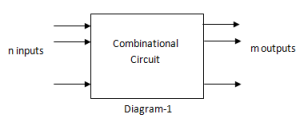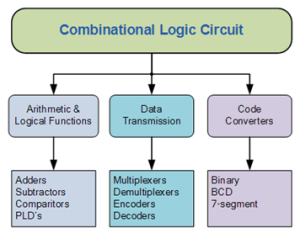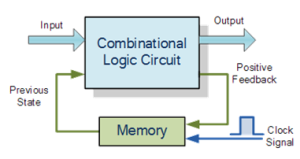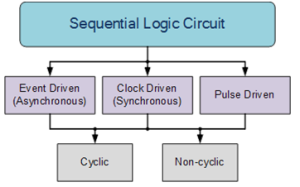# Combinational Logic Circuit vs. Sequential Logic Circuit

## Combinational Logic Circuit vs. Sequential Logic Circuit

Logic Circuits can be divided into two types:

1. Combinational Logic Circuit
2.  Sequential Logic Circuit

In this article we will discuss Combinational Logic Circuit vs. Sequential Logic Circuit.

### Combinational Logic Circuits

Combinational Logic circuit contains logic gates where its output is determined by the combination of the current inputs, regardless of the output or the prior combination of inputs. Basically, combinational circuit can be depicted by diagram-1 below:In a combinational logic circuit, the value of the outputs depends only on the current values of the inputs. The circuit has no memory or feedback loops, and the output does not depend on the history of the inputs.

Examples of Combinational circuits are adder, Subtractor, encoder, decoder, parallel adder, multiplexer and de-multiplexer.

Combinational Logic Circuits are made up from basic logic gates such as NAND, NOR or NOR gates that are combined or connected together to produce more complicated switching circuits. These logic gates are the building blocks of Combinational Logic Circuits.

Combinational logic circuits can be very simple or very complicated and any combinational circuit can be implemented with only NAND and NOR gates as these are classed as universal gates.

The three main ways of specifying the function of a combinational logic circuit are:

1. Boolean Algebra:  This forms the algebraic expression showing the operation of the logic circuit for each input variable either True or False that results in a logic “1” output.
2. Truth Table: A truth table defines the function of a logic gate by providing a concise list that shows all the output states in tabular form for each possible combination of input variable that the gate could encounter.
3. Logic Diagram: This is a graphical representation of a logic circuit that shows the wiring and connections of each individual logic gate, represented by a specific graphical symbol, that implements the logic circuit.

#### Classification of Combinational Logic CircuitsFig.2

### Sequential Logic Circuit

Unlike combinational logic circuits whose output depends upon the signals being applied to the inputs at that time, Sequential Logic circuits have some form of  memory built in to them so that they are able to take into account their previous input state as well as those at present.

In other words, the output state of a “sequential logic circuit” is a function of the following three states, the “present input”, the “past input” and/or the “past output”.

Sequential Logic circuits remember these conditions and stay fixed in their current state until the next clock signal changes one of the states, giving sequential logic circuits “Memory”.

Sequential logic circuits are generally termed as two state or bistable devices which can have their output or outputs set in one of two basic states, a logic level “1” or a logic level “0” and will remain “latched” indefinitely in this current state or condition until some other input trigger pulse or signal is applied which will cause the bistable to change its state once again.

#### Sequential Logic RepresentationFig.3

Simple sequential logic circuits can be constructed from standard bistable circuits such as: Flip-flops, Latches and Counters and which themselves can be made by simply connecting together universal NAND Gates and/or NOR Gates in a particular combinational way to produce the required sequential circuit.

#### Classification of Sequential Logic

Bistable latches and flip-flops are the basic building blocks of sequential logic circuits.

Sequential logic circuits can be constructed to produce either simple edge-triggered flip-flops or more complex sequential circuits such as storage registers, shift registers, memory devices or counters.

Either way sequential logic circuits can be divided into the following three main categories:

1. Event Driven – Asynchronous circuits that change state immediately when enabled.
2. Clock Driven – synchronous circuits that are synchronized to a specific clock signal.
3. Pulse Driven – Which is a combination of the two that responds to triggering pulses.Fig.4

In addition to  the two logic states i.e. logic level “1” and logic level “0”, a third element is introduced which separates sequential logic circuits from their combinational logic counterparts, is TIME.

Sequential logic circuits return back to their original steady state once reset and sequential circuits with loops or feedback paths are said to be “cyclic” in nature.

A sequential circuit in which changes occur only on the application of a clock signal makes it asynchronous, otherwise the circuit is asynchronous and depends upon an external input.